# Intro to R Programming

## Agenda

• The R User Interface
• Command Line Interface
• Objects/Variables
• Atomic Vector
• Built-in Functions

## The R User Interface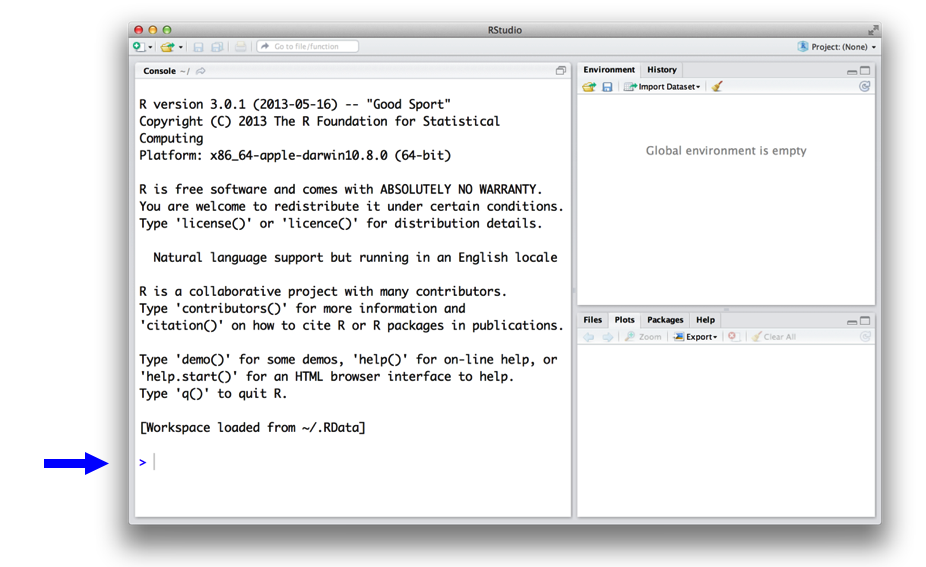## Interacting with R

• You can use R interactively through its command line interface (CLI) or console:
• enter a command
• R executes the command
• get a printed response
• (repeat)
• You can use R in batch mode:
• give R a script: a list of commands
• R executes the commands
• get result(s) displayed on screen or stored in files: text, tables, graphics, and more

## Command Line Interface

``1 + 1``
``##  2``
``4 * 1.3``
``##  5.2``
``4 / 7``
``##  0.5714286``

## Command Line Interface

``4 / 7``
``##  0.5714286``
• ``: this line begins with the first value in the result.
``50:89``
``````##   50 51 52 53 54 55 56 57 58 59 60 61 62 63 64 65 66 67 68 69 70 71 72 73 74
##  75 76 77 78 79 80 81 82 83 84 85 86 87 88 89``````
• `:` returns every integer between two integers.
• The result returned is of type vector.

## Live Coding Exercise!

• Choose any number and add 2 to it.
• Multiply the result by 3.
• Subtract 6 from the answer.
• Divide what you get by 3.

Let’s code!

## Live Coding Exercise

``14 + 2``
``##  16``
``16 * 3``
``##  48``
``48 - 6``
``##  42``
``42 / 3``
``##  14``

## R Objects/Variables

• Object/variable is a name that you can use to call up stored data.
• To assign/store value to an object/variable, use `=` or `<-` notation.
``````a <- 1
a``````
``##  1``
``a + 2``
``##  3``

## R Objects/Variables

``````die <- 1:6
die``````
``##  1 2 3 4 5 6``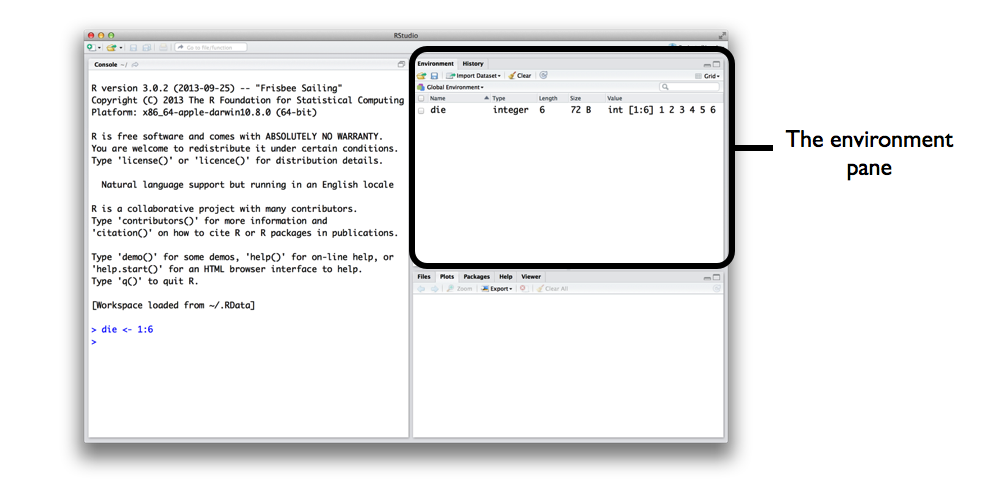## R Objects/Variables

Good names Names that cause errors
a 1trial
b \$
FOO ^mean
my_var 2nd
.day !bad
• R is case-sensitive.
• `name` and `Name` will refer to different objects.

## R Vector

• In R, vector includes: atomic vector and list.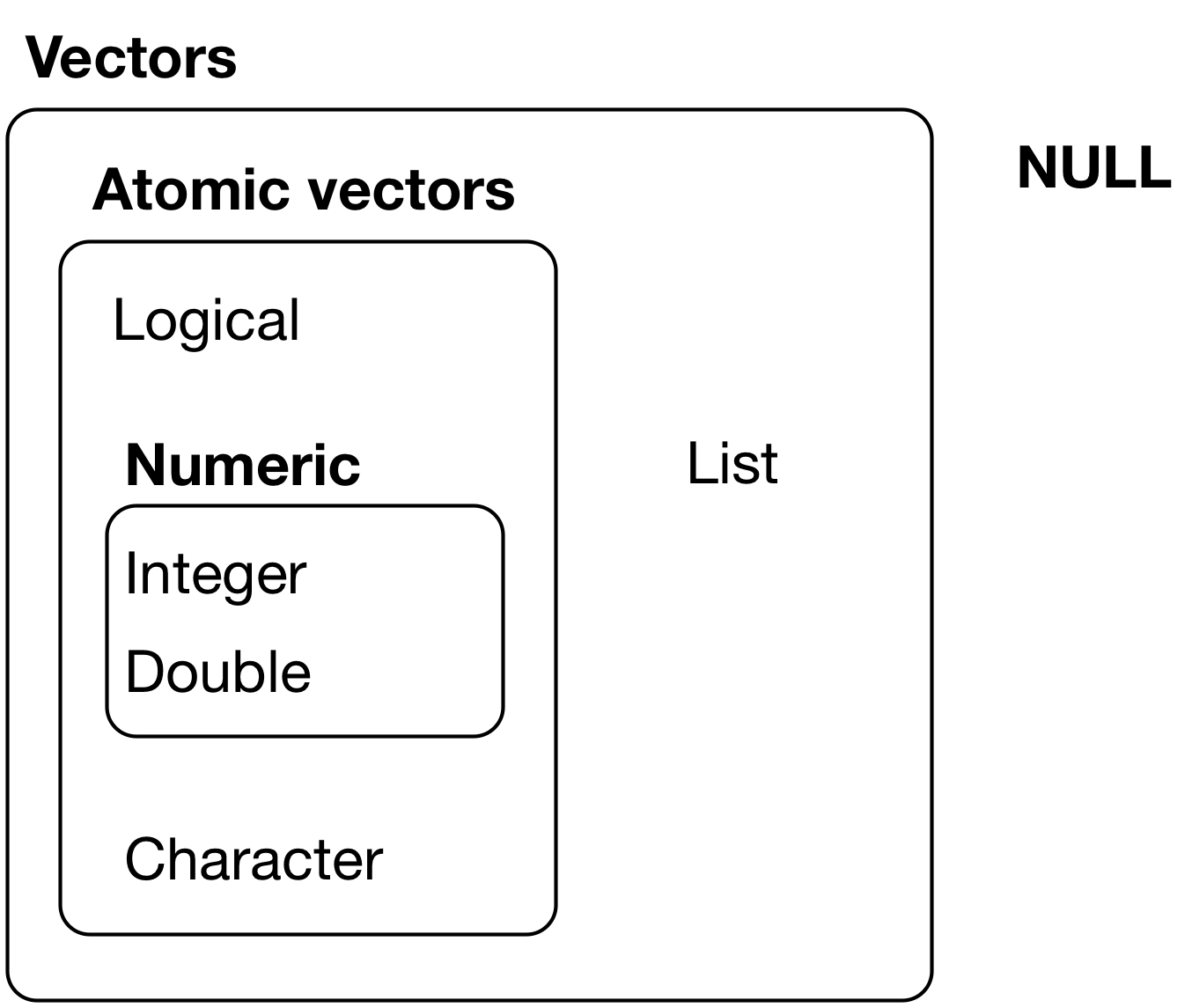## R Vector

• Vector stores its values as a one-dimensional array.
• Vector index starts at 1 (unlike in other programming languages).
``die``
``##  1 2 3 4 5 6``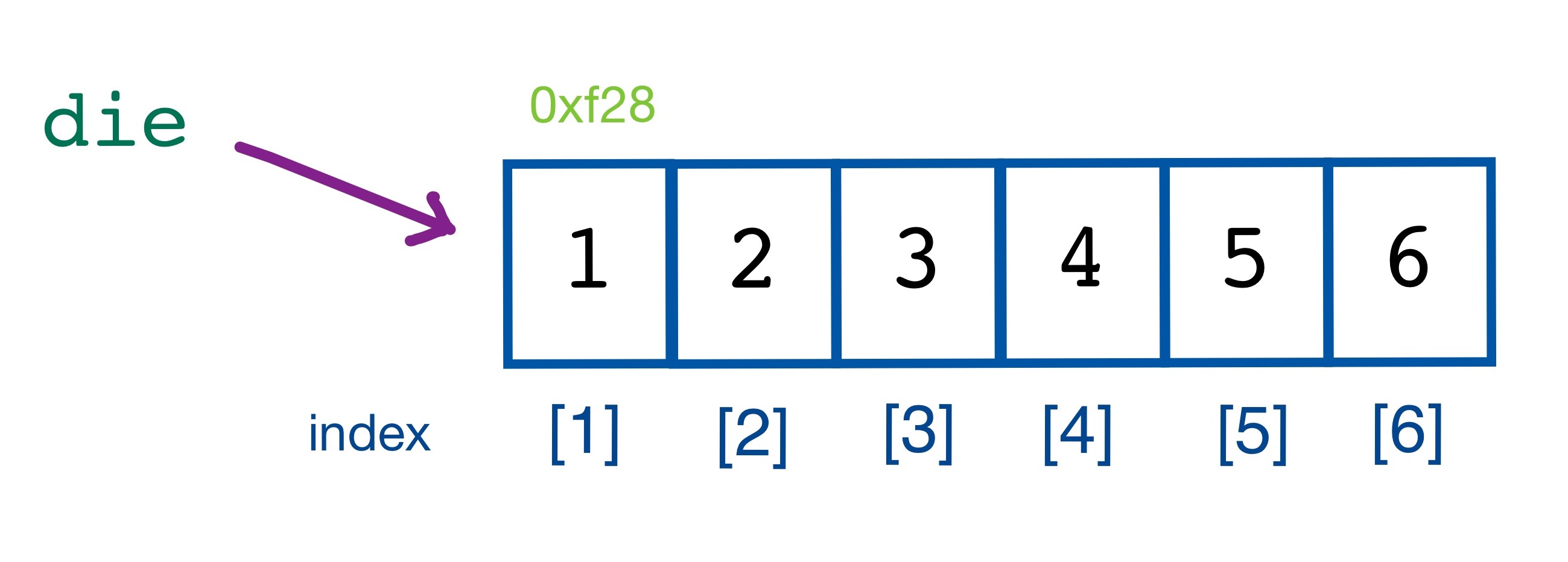## Atomic Vector

• Each atomic vector can only store one type of data.
• `c` is a function that concatenates scalars into a vector.
• Use `c` to create a vector from individual values.
``````fruits <- c("banana", "apple", "orange", "strawberry", "pineapple")
fruits``````
``##  "banana"     "apple"      "orange"     "strawberry" "pineapple"``
``````long_vector <- c(fruits, c("peach", "mango"))
long_vector``````
``````##  "banana"     "apple"      "orange"     "strawberry" "pineapple"
##  "peach"      "mango"``````

## Atomic Vector

``````logic <- c(TRUE, FALSE, TRUE, TRUE)
logic``````
``##   TRUE FALSE  TRUE  TRUE``
``logic <- c(True, False, True, True)``
``## Error in eval(expr, envir, enclos): object 'True' not found``
``````mixed <- c(TRUE, 2, "Hello")
mixed``````
``##  "TRUE"  "2"     "Hello"``
``````mixed <- c(TRUE, 2)
mixed``````
``##  1 2``

## Atomic Vector

• Use index to access each element of a vector.
``fruits``
``##  "banana"     "apple"      "orange"     "strawberry" "pineapple"``
``fruits``
``##  "banana"``
``fruits``
``##  "apple"``
``fruits[1:3]``
``##  "banana" "apple"  "orange"``

## Atomic Vector

• R uses element-wise execution.
• When you manipulate a set of numbers, R will apply the same operation to each element of the set.
``die - 1``
``##  0 1 2 3 4 5``
``die / 2``
``##  0.5 1.0 1.5 2.0 2.5 3.0``
``die * die``
``##   1  4  9 16 25 36``
``die > 3``
``##  FALSE FALSE FALSE  TRUE  TRUE  TRUE``

## Atomic Vector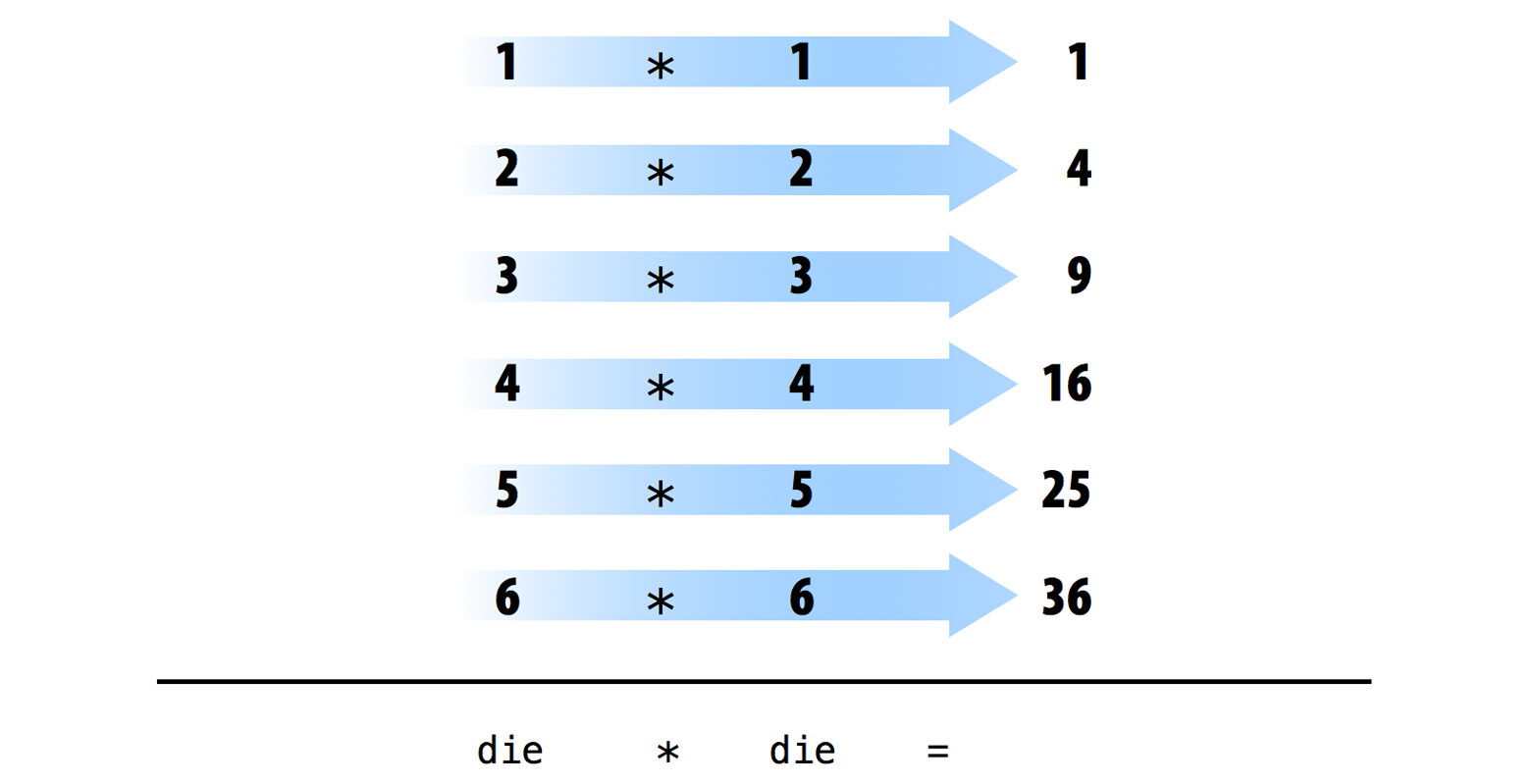## Atomic Vector

• Things get complicated when we give R two vectors of unequal lengths.
• In this case, R will repeat the shorter vector until it is as long as the longer vector, and then do the math.
``1:2``
``##  1 2``
``die``
``##  1 2 3 4 5 6``
``die + 1:2``
``##  2 4 4 6 6 8``